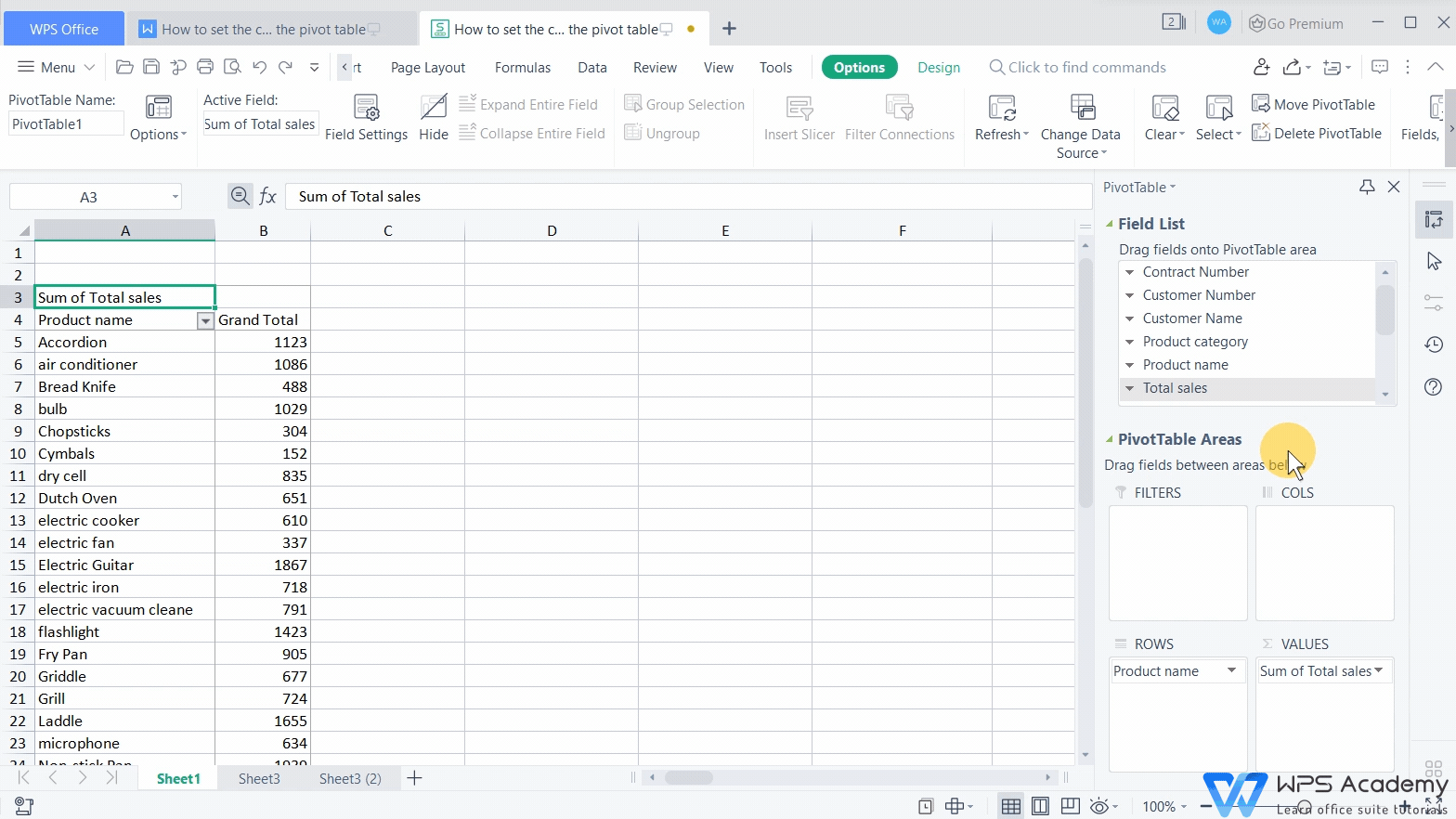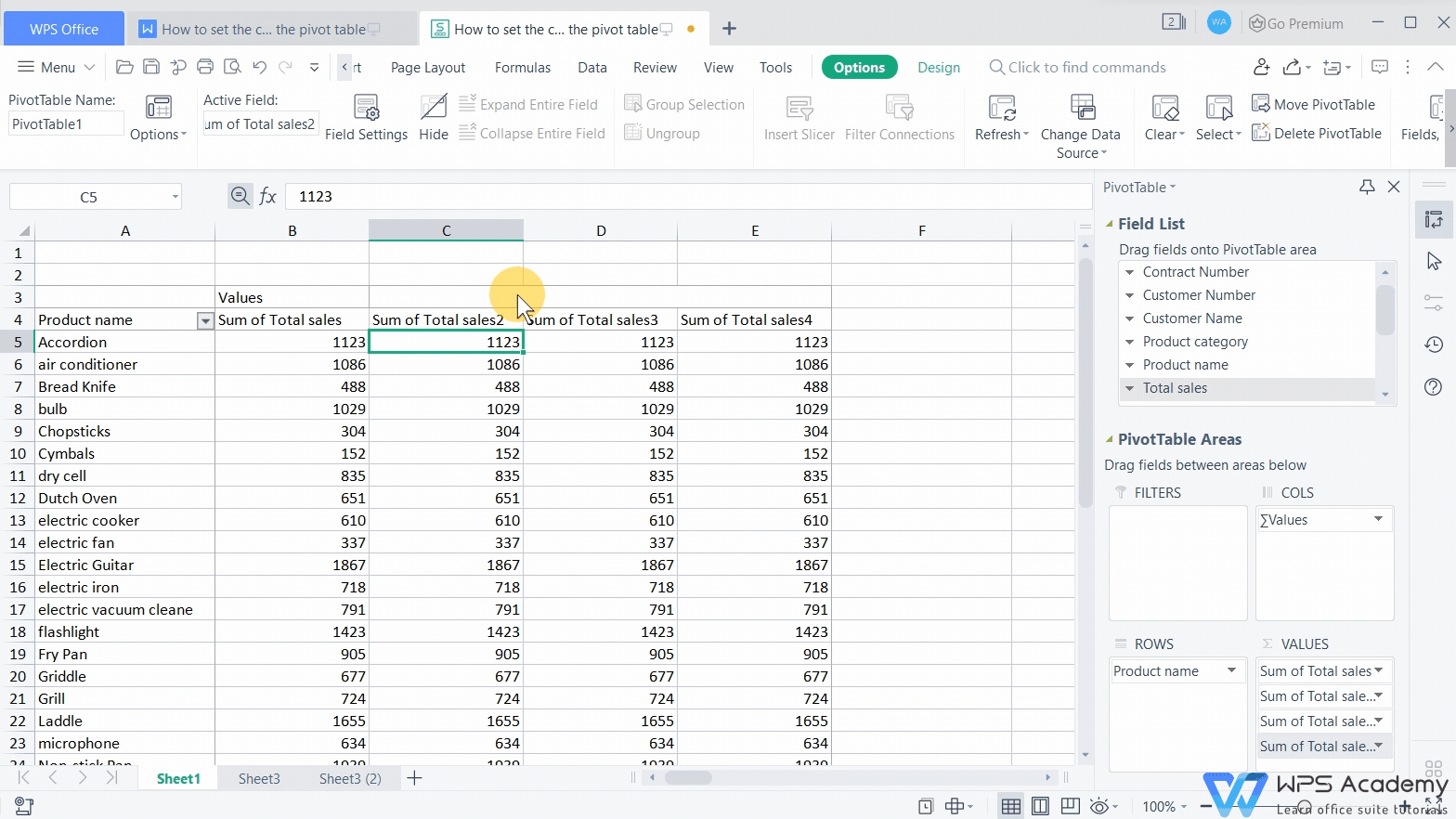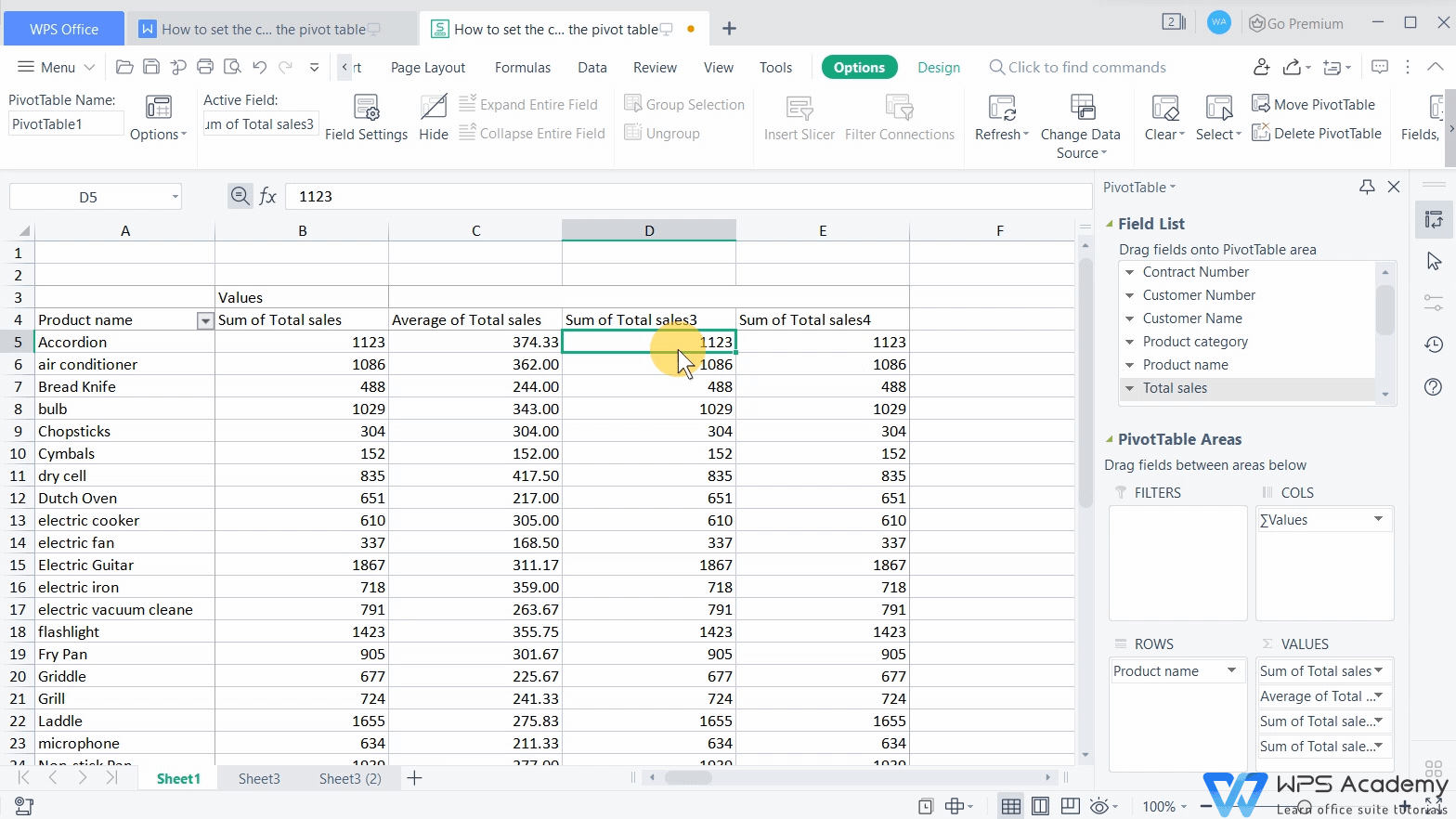# How to set the calculated field of the pivot table

Uploaded time: September 10, 2021 Difficulty: Beginner# How to set the calculated field of the pivot table

We often need to use a pivot table to calculate sales in the work of commodity sales. So how can we quickly count the sales by setting the calculated fields of the pivot table?

Take this pivot table as an example. As is shown in this pivot table, if we want to count the total sales, average sales, maximum sales, and minimum sales of each product, we only need to enter the fields in the pivot table to set it up.

First, we need to drag the Total sales field to the VALUES list box three times in a row, then the Sum of Total sales 2, Sum of Total sales 3 and Sum of Total sales 4 will show up in the pivot table.1. Click any cell under the field Sum of Total sales 2 with the mouse.

2. Click the Field Settings button, and select the Average option in the pop-up dialog box.

3. Now the value below will change to the average value.

4. To make the form looks better, we select the cell area under the field Average of Total sales, right-click, and select Format Cells in the shortcut menu.

5. Then select Number in the Format Cells dialog box, and keep two decimal places.

6. Finally, click OK.In the same way, we can set the Sum of Total sales 3 as the maximum value. Also, we can set the Sum of Total sales 4 as the minimum value.Through the pivot table, we can quickly calculate the needed data in the sales table. Did you get it?

To be an office excel advancers, you could learn how to use WPS Office Spreadsheet online in WPS Academy.